Using Turf.js with Leaflet to calculate the distance between to points

This article assumes the reader has basic knowledge of JavaScript and Leaflet. Details about the code will be given only on the most important parts of the code, especial the parts where Turf.js is involved and the parts that create interaction with the map. For more information about Turf.js, visit the official page here.

To run the example above on your own computer, copy/paste the code below to a txt file and save it as html.

To view the whole code, scroll down in the grey area.

Hidden

To calculate the distance between two points, Turf uses the Haversine formula, a formula that takes in the account the global curvature of the Earth.

The lines below include the Leaflet JavaScript file and the Turf.js library to the map.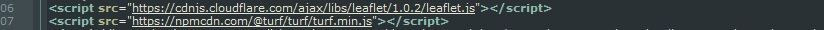The next lines of code create the map and the static point to witch all the distances are calculated. This is the standard
approach of creating a map and a GeoJSON point in Leaflet, add there for it will not be disused here. There are many tutorials
on Leaflet main page that show how to create a map instance and how to add different geo-spatial data on the map.

The onEachFeatureCoord function makes it easyer to calculate the distance between the Red Marker and each Blue Marker
added on the map. Next, the map.on('click', function)(e) adds the marker on map when the map is clicked and makes the
marker dragable. The function marker.on('drag', function(e)) gets the coordinates of each Blue Marker when is draged/moved
on the map, to variables lat lng.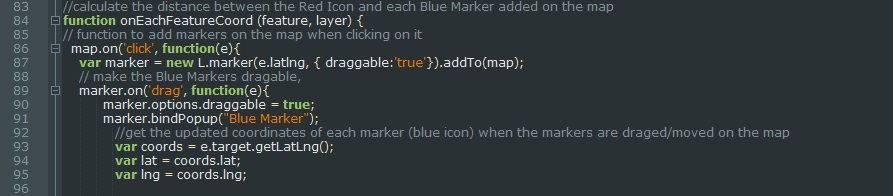The sintax of turf.distance module is: turf.distance (from, to, [units=kilometers]). Units can be as well degrees, radians,
miles. The next group of code inside the "getNewDistance" function, calculates the distance between the Red Marker and
each draged/moved Blue Marker and saves the result in dist variable. In this case, the code line that calculates the distance
is: turf.distance(redIcon.features[i].geometry.coordinates, [lng,lat], units). The from parameter is in this case
redIcon.features[i].geometry.coordinates, the to parameter is [lng,lat]. The units parameter is declared outside the
onEachFeatureCoord function, and takes the value kilometers.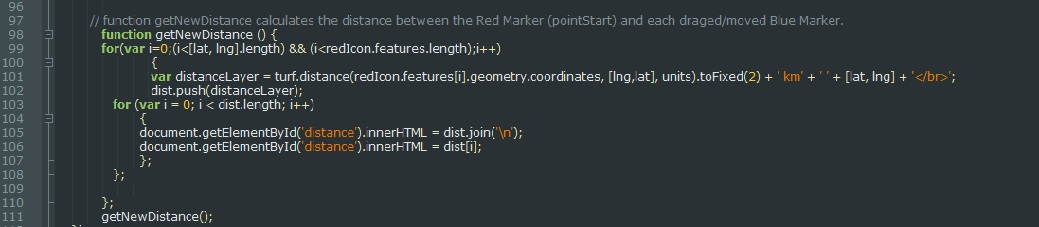The next group of code converts the data inside the variable named "marker" in GeoJSON format. The conversion is done
to make it easier to select the coordinates of each marker added to the map.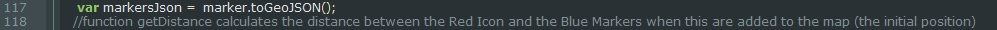The function "getDistance" calculates the distance between the Red Icon and the Blue Markers when this are added to the
map (the initial position).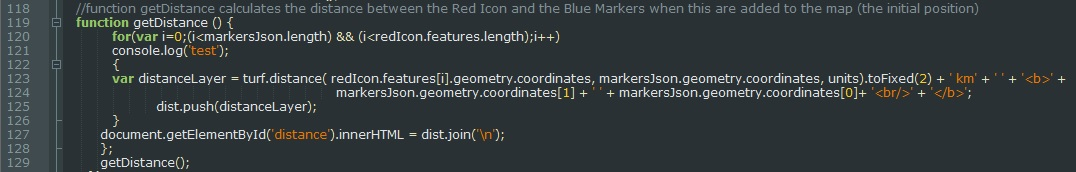The content of onEachFeatureCoord is passed to the oneEachFeature's option of the GeoJSON redIcon.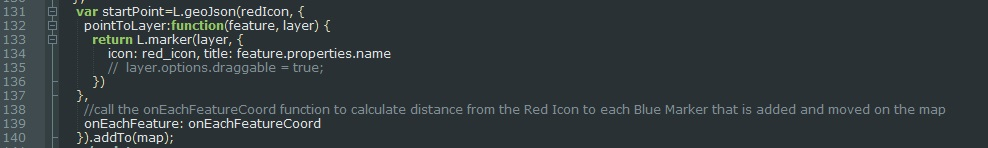For questions and suggestions related to this article, send e-mail at corina@mapwizard.eu.

Hidden